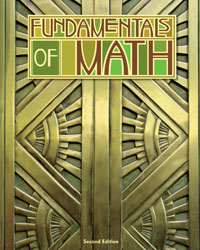## BJU Fundamentals of Math Student Text (2nd ed.)

• ₱2,410.00

Fundamentals of Math Student Text (2nd edition) covers concepts such as whole numbers, decimals, number theory, fractions, and an introduction to algebra and geometry. Each chapter contains "Dominion thru Math" exercises that apply mathematics to everyday life, "Mind over Math" and "Problem Solving" sections that promote critical thinking skills, and a chapter review section.

• Edition - 2nd (locally printed with permission)
• Item Code - 218933

We Also Recommend